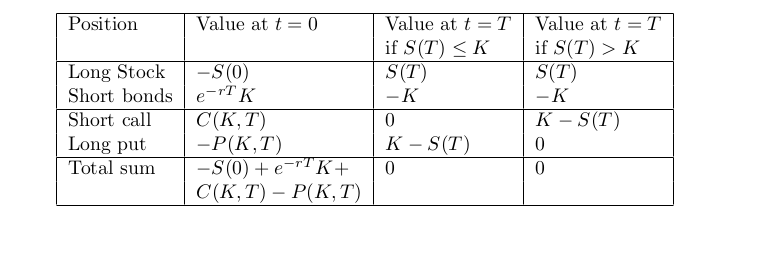# Put-Call-Parity

We'll explore a relationship between the price of a European call option and a European put option that both have the same underlier, expiry, and strike price. This knowledge can help you identify arbitrage opportunities in an options market if the put-call parity isn’t maintained, as well as pin down prices for a put given a call price or vice-versa.
We’ll first discuss some aspects of interest rates that we will need in this and future articles.

## Compounding Interest Rates

Simple interest rates do not involve the compounding of money. For example, if $100 is invested at an annual interest rate of 6 percent, it grows to$106 a year from now. Compound interest is when interest is earned on the principal plus any accrued interest. For example, if the compounding interval is 1 month, with an annual rate of 6 percent, $100 grows to 100 + 6/12 =$100.5 in a month. In a year, there are 12 such compounding periods, so it grows to $100*(1+6/12)¹² =$106.16.
In general, if the interest rate is r (in decimals, so 6 percent means r = 0.06), and there are m compounding periods in a year, the principal grows to (1+r/m)ᵐ times the principal in a year. If the interest is continuously compounded, that is, we let m become very large, and the expression (1+r/m)ᵐ approaches the exponential , where e is a special mathematical constant that is roughly 2.71828. In our previous example, exp(0.06)= 1.0618. In general, if continuous compounding at the constant rate of r occurs until time t, we have exp(rt).

## Zero-Coupon Bonds

A zero-coupon bond (ZCB) with face value (par value) K and maturity T is an interest-bearing instrument in which an investor can pay a discounted price B_0 relative to par value today to purchase the right to earn K at time T. This is also sometimes called a money market account. Assuming a constant continuously compounded risk-free rate r, the price of a ZCB today is B_0 = K*exp(-rT).

## Put-Call Parity

Now to return to the previously scheduled option strategy content. Something magical happens when we buy (long) a call option, and write (short) a put option, with the same strike price and expiration:In this case, we have a portfolio consisting of a long call option with a strike price of 100 and a short put option with a strike price of 100 (shown in the left column), contrasted with a portfolio consisting of that stock with a short bond with a future liability of 100 (shown on the right column). Notice that when added, both portfolios give the same payoff. We can write this as:
$\text{Long call + short put = long stock + short bond}$
Rearranging the terms in this equation also yields another interesting relationship:
$\text{Long stock + short call = short put + long bond}$
In words, a covered call = cash secured put, where the put and call have the same strike and expiration.
We can also represent this using a table:In the above portfolio, we’ve moved the long call and short put on the left-hand side of the equation above to the right-hand side. Notice that at time t = T, the portfolio is always worth S(T)-K + K -S(T) = 0, regardless of whether S(T) is greater than or less than K. So, the portfolio must be worth 0 initially (at time t=0). Otherwise, we have an arbitrage opportunity. This allows us to write put-call parity in the following form, which is the same as the relationship obtained from the payoff diagram above (note that a long call has the negative payoff of a short call):
$C(K, T) — P(K, T) = S(0)-exp(-rT)K$
Let’s consider an example to see how this relationship can be used:
• A stock costs S(0) at t = 0. For example, let this be $112.5. We represent its future price at time t= T as S(T) • Let the current compounded interest rate be 5 percent a year, and let T be one year so that exp(-0.05*1) = 0.95. Note that the shorted bond needs to be repaid with the amount K = 100 after one year. Short selling the bond today (i.e., borrowing it to sell) gives us 100*exp(-0.05*1) • The put costs p today. (If S(T) < K at expiration, then the put pays K-S(T)). • The call costs c today. (If S(T) > K at expiration, the short call is worth K-S(T)) • Suppose the price of a put today is$2.5. Now we can determine the arbitrage-free price of the call.
Set
$c = S(0) - exp(-rT)K + p = 112.5 – 0.95*100 + 2.5 = 20.$
This implies the arbitrage-free price for the call option is \$20.